# Paired comparison model

(diff) ← Older revision | Latest revision (diff) | Newer revision → (diff)

The basic paired comparison model was presented by R.A. Bradley and M.E. Terry in [a1] (cf. also Bradley–Terry model). The paired comparison experiment hasobjectswith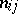comparisons of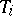and,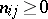,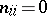,,. The model postulates the existence of treatment parameters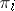for(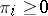),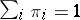, such that the probability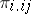of selecting, when compared with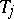, is equal to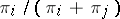. A model for incorporating ties has been introduced by P.V. Rao and L.L. Kupper in [a2] and by R.R. Davidson in [a3].

Rao and Kupper introduced a threshold parameter,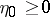, which is interpreted as the threshold of sensory perception for the judge. They model the probabilities of preferenceand of no preference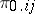asandwhere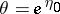. For, the Rao–Kupper model coincides with the Bradley–Terry model. In [a4], R.J. Beaver and D.V. Gokhale have generalized the model to incorporate within-pair order effects. They assume the existence of parameters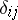,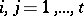,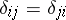, associated with the pair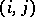, such that the preference probabilities for the ordered pairare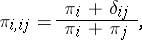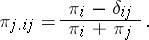Thurstone's model (see [a5]) assumes that a person receives in response toa sensationwhich is normally distributed with locationon a subjective continuum (cf. also Normal distribution). A special case gives that the probability thatis preferred tois equal to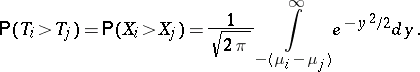If the normal density function is replaced by the logistic density function, the model is equal to the Bradley–Terry model with. H. Stern has considered, [a6], models for paired comparison experiments based on comparison of gamma random variables. Different values of the shape parameter yield different models, including the Bradley–Terry model and the Thurstone model. Likelihood methods can be used to estimate the parameters of the models. The likelihood equations must be solved with iterative methods.

It is also possible to fit response surfaces in paired comparison experiments (see, e.g., [a7], [a8]). Mostly it is assumed that the parameters,, are functions of continuous variablessuch that the formulated model is linear in the unknown parameters. If such a model is formulated, then it is possible to discuss the question of optimal design in paired comparison experiments. Many criteria for optimal design depend on the variance-covariance matrix of the estimators for the unknown parameters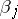. However, the asymptotic variance-covariance matrix itself depends on the unknown parameters (see, e.g., [a7], [a8]). A. Springall has defined, [a7], so-called analogue designs. These are designs in which the elements of the paired comparison variance-covariance matrix are proportional to the elements of the classical response surface variance-covariance matrix with the same design points.

In order to find designs, E.E.M. van Berkum has assumed, [a8], that the parametersare all equal. In that case the variance-covariance matrix is proportional to the variance-covariance matrix for the estimators in an ordinary linear model and general optimal design theory can be applied (-optimality,-optimality, equivalence theorem). He also gives optimal designs for various factorial models.

There is much literature on paired comparison experiments and related topics such as generalized linear models, log-linear models, weighted least squares and non-parametric methods. A bibliography up to 1976 is given in [a9]. The state of the art as of 1976 is given in [a10], and as of 1992 in [a11].

How to Cite This Entry:
Paired comparison model. Encyclopedia of Mathematics. URL: http://encyclopediaofmath.org/index.php?title=Paired_comparison_model&oldid=14585
This article was adapted from an original article by E.E.M. van Berkum (originator), which appeared in Encyclopedia of Mathematics - ISBN 1402006098. See original article